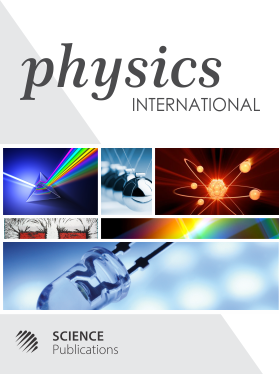Frequency: Continuous
ISSN: 1948-9803 (Print)
ISSN: 1948-9811 (Online)
Research Article Open Access

# THE NUMERICAL VALUES OF THE FUNDAMENTAL PARAMETERS OF THE MODEL OF SELFVARIATIONS AND THE AGE OF THE UNIVERSE

Emmanuil Manousos1
• 1 National and Kapodistrian University of Athens, Greece
Physics International
Volume 5 No. 1, 2014, 8-14

DOI:

Submitted On: 18 February 2014 Published On: 20 March 2014

How to Cite: Manousos, E. (2014). THE NUMERICAL VALUES OF THE FUNDAMENTAL PARAMETERS OF THE MODEL OF SELFVARIATIONS AND THE AGE OF THE UNIVERSE. Physics International, 5(1), 8-14. https://doi.org/10.3844/pisp.2014.8.14

## Abstract

The law of selfvariations determines quantitatively a slight increase of the rest masses and the electric charges of material particles as a common cause of quantum and cosmological phenomena. At cosmological scales the law of selfvariations can be expressed by two similar differential equations for the rest mass and the electric charge respectively. These equations contain information and justify the totality of the cosmological data. Solving these equations, we introduce two parameters k, A for the rest mass and another two k1, B, for the electric charge. Knowledge of the numerical values of these four parameters suffices for the accurate determination of the predictions of the law of selfvariations at cosmological scales. In the present article we determine the intervals in which these fundamental parameters obtain their values. As an aside, the conclusion emerges that the age of the Universe is far larger than the one predicted by the Standard Cosmological Model. A very long time of evolution is predicted until the Universe takes the form in which we observe it today.

• 1,622 Views